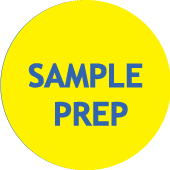# Linear Calibration curve

Abstract Quantitative analysis is based on least squares calibration curves. Calculation of a least squares curve assumes that the standard deviation is constant over the calibration range and that the uncertainty of the response is greater than the uncertainty in the reference concentrations. The uncertainty in calculated concentrations stems both from uncertainty in the measured response for the unknown and from the uncertainty inherent in the construction of the calibration curve. If a method’s calibration curve is linear and stable, recalibration can be simplified greatly. Note that correlation coefficients do not give sufficient information to characterize a calibration curve.

KeywordsLeast squares, Calibration curve, Uncertainty, Slope, Intercept, Standard deviation, Confidence interval, Correlation coefficient (Rc), Linearity, Random error, Linear dynamic range